Graphing Quadratic Functions (page 1 of 4)

Sections: Introduction, The meaning of the leading coefficient / The vertex, ExamplesThe general technique for graphing quadratics is the same as for graphing linear equations. However, since quadratics graph as curvy lines (called "parabolas"), rather than the straight lines generated by linear equations, there are some additional considerations.

The most basic quadratic is y = x2. When you graphed straight lines, you only needed two points to graph your line, though you generally plotted three or more points just to be on the safe side. However, three points will almost certainly not be enough points for graphing a quadratic, at least not until you are very experienced. For example, suppose a student computes these three points: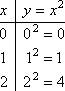Then, based only on his experience with linear graphs, he tries to put a straight line through the points.

 incorrect graph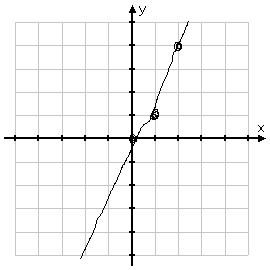He got the graph wrong. You, on the other hand, are more careful.

 You find many points: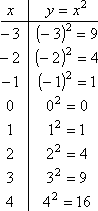That last point has a rather large y-value, so you decide that you won't bother drawing your graph large enough to plot it.

 But you plot all the other points:Even if you'd forgotten that quadratics graph as curvy parabolas, these points will remind you of this fact.

You draw a nicely smooth curving line passing neatly through the plotted points:

 correct graph of y = x2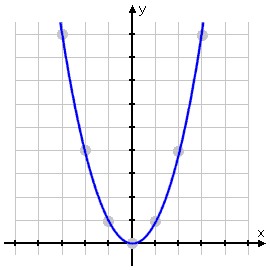Unlike the careless student, you just got the graph right.

Some students will plot the points correctly, but will then connect the points with straight line segments, like this:

 incorrect "segment" graph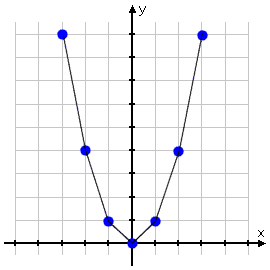This is not correct. You do still need a ruler for doing your graphing, but only for drawing the axes, not for drawing the parabolas. Parabolas graph as smoothly curved lines, not as jointed segments.

Top  |  1 | 2 | 3 | 4  |  Return to Index  Next >>